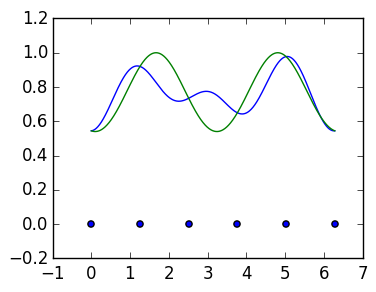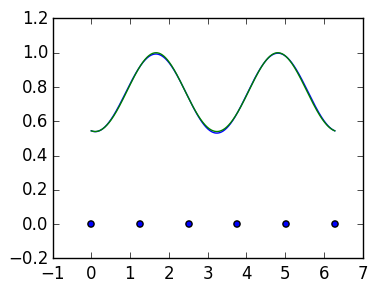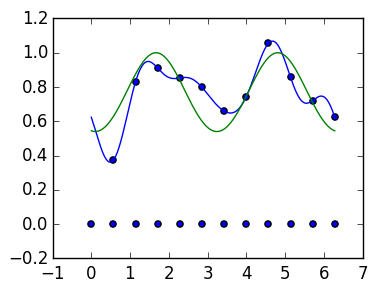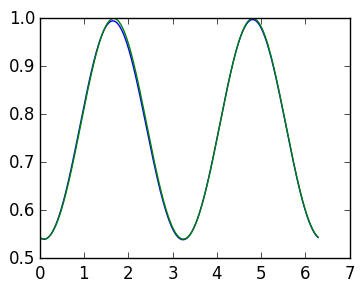# Lecture 32: The Discrete Fourier Transform¶

In this lecture we introduce the Discrete Fourier Transform (DFT), which is a matrix that maps from the function values $[f(\theta_1),\ldots,f(\theta_n)]$ to the discrete Fourier coefficients $[\hat f_0^n,\ldots,\hat f_{n-1}^n]$. We will also introduce the inverse DFT, that maps back. Understanding the relationship between the DFT and its inverse will show why the discrete Fourier expansion interpolates the data.

The first step is to split the discrete Fourier expansion into two steps:

1. Evaluate the function at the points $\theta_1,\ldots,\theta_n$ (func_to_vals)
2. Transform from the values to the coefficients $[\hat f_0^n,\ldots,\hat f_{n-1}^n]$ (dft)

The following routines recast the dft command as a map from a Vector of function samples to the coefficients. This is equivalent to using the Trapezium rule (trap last lecture).

In :
using PyPlot

function func_to_vals(f::Function,n)
θ=linspace(2π/n,2π,n)
map(f,θ)
end

function dft(vals::Vector)   # assume α=0,β=n-1
n=length(vals)
cfs=zeros(Complex128,n)

θ=linspace(2π/n,2π,n)

for k=0:n-1
for j=1:n
cfs[k+1]+=vals[j]*exp(-im*k*θ[j])/n
end
end

cfs
end

f=θ->cos(cos(θ-0.1))

n=5
vals=func_to_vals(f,n)
fc=dft(vals)

Out:
5-element Array{Complex{Float64},1}:
0.765198+0.0im
0.00226385+0.000976271im
-0.112613+0.0228279im
-0.112613-0.0228279im
0.00226385-0.000976271im

We can now evaluate the approximate Fourier expansion. Note that we equal the original function, as specified by the data vals, at $\theta_j = {2 \pi j \over n}$:

In :
# sum an approximate Fourier expansion
function fours(fc::Vector,α,β,θ)
ret=0.+0.im

for k=α:β
ret += fc[k-α+1]*exp(im*k*θ)
end
ret
end

g=linspace(0.,2π,1000)

plot(g,real(map(θ->fours(fc,0,n-1,θ),g)))
plot(g,f(g))

scatter(linspace(0.,2π,n+1),zeros(n+1))Out:
PyObject <matplotlib.collections.PathCollection object at 0x30aa34690>

## The Inverse DFT¶

Let's consider now the inverse problem: evaluating the discrete Fourier expansion

$$p(\theta) = \sum_{k=0}^{n-1} \hat f_k^n e^{i k \theta}$$

at the points $\theta_j$. This relationship is straightforward by constructing a Vandermonde-like system:

$$\begin{pmatrix} p(\theta_1) \cr p(\theta_2) \cr \vdots \cr p(\theta_n) \end{pmatrix} = \begin{pmatrix} 1 & e^{i \theta_1} & \cdots & e^{i (n-1) \theta_1} \cr 1 & e^{i \theta_2} & \cdots & e^{i (n-1) \theta_2} \cr \vdots & \vdots & \ddots & \vdots \cr 1 & e^{i \theta_{n}} & \cdots & e^{i (n-1) \theta_n} \end{pmatrix} \begin{pmatrix} \hat f_0^{n} \cr \hat f_1^{n} \cr \vdots \cr \hat f_{n-1}^{n} \end{pmatrix}$$

We define this matrix as the inverse DFT, which we can construct as iDFT:

In :
function iDFT_matrix(n)
iDFT=zeros(Complex128,n,n)
θ=linspace(2π/n,2π,n)

for j=1:n,k=0:n-1
iDFT[j,k+1] = exp(im*k*θ[j])
end
iDFT
end

iDFT=iDFT_matrix(n)

norm(iDFT*fc-vals)

Out:
3.1582658042774047e-16

By definition, the inverse of iDFT gives a function that interpolates the data:

In :
DFT_as_inv=inv(iDFT)

norm(DFT_as_inv*vals-fc)

Out:
1.485071973177909e-16

## DFT and interpolation¶

We can also reinterpret the dft command as a matrix:

$${1 \over n} \begin{pmatrix} 1 & 1 & \cdots & 1 \cr e^{-i \theta_1} & e^{-i \theta_2} & \cdots & e^{-i \theta_n} \cr \vdots & \vdots & \ddots & \vdots \cr e^{-i (n-1) \theta_1} & e^{-i (n-1) \theta_2} & \cdots & e^{-i (n-1) \theta_n} \end{pmatrix} \begin{pmatrix} f(\theta_1) \cr f(\theta_2) \cr \vdots \cr f(\theta_n) \end{pmatrix} = \begin{pmatrix} \hat f_0^{n} \cr \hat f_1^{n} \cr \vdots \cr \hat f_{n-1}^{n} \end{pmatrix}$$

We construct it as the matix DFT:

In :
function DFT_matrix(n)
DFT=zeros(Complex128,n,n)
θ=linspace(2π/n,2π,n)

for j=1:n,k=0:n-1
DFT[k+1,j] = exp(-im*k*θ[j])/n
end
DFT
end

DFT=DFT_matrix(n)

norm(DFT*vals-fc)

Out:
5.003707553108401e-17

In other words, DFT is the inverse of iDFT:

In :
norm(DFT*iDFT-I)

Out:
3.582159729514101e-16

This follows from the fact that

$$\sum_{j=1}^n e^{i k \theta_j} = \begin{cases} n & \hbox{k is a multiple of n} \cr 0 & \hbox{otherwise}\end{cases}$$

and the formula:

$$\begin{pmatrix} 1 & 1 & \cdots & 1 \cr e^{i \theta_1} & e^{i \theta_2} & \cdots & e^{i \theta_n} \cr \vdots & \vdots & \ddots & \vdots \cr e^{i (n-1) \theta_1} & e^{i (n-1) \theta_2} & \cdots & e^{i (n-1) \theta_n} \end{pmatrix} \begin{pmatrix} 1 & e^{i \theta_1} & \cdots & e^{i (n-1) \theta_1} \cr 1 & e^{i \theta_2} & \cdots & e^{i (n-1) \theta_2} \cr \vdots & \vdots & \ddots & \vdots \cr 1 & e^{i \theta_{n}} & \cdots & e^{i (n-1) \theta_n} \end{pmatrix} = \begin{pmatrix} n & \sum_{j=1}^n e^{i \theta_j} & \sum_{j=1}^n e^{i 2 \theta_j} & \cdots & \sum_{j=1}^n e^{i (n-1) \theta_j} \cr \sum_{j=1}^n e^{-i \theta_j} & n & \sum_{j=1}^n e^{i \theta_j} & \cdots & \sum_{j=1}^n e^{i (n-1) \theta_j} \cr \vdots & \vdots & \vdots & \ddots & \vdots \cr \sum_{j=1}^n e^{-i (n-1) \theta_j} & \sum_{j=1}^n e^{-i (n-2) \theta_j} & \sum_{j=1}^n e^{-i (n-3) \theta_j} & \cdots & n \end{pmatrix} = n I$$

Note that the DFT and the iDFT are conjugate transposes (within a factor of n):

DFT = n*iDFT'

(Recall: ' is the conjugate transpose while .' is the transpose. These are equivalent for real matrices.) This means that the DFT and iDFT are a scaled unitary matrix:

In :
Q=iDFT/sqrt(n)

norm(Q'*Q-I)

Out:
3.666681001976133e-16

## Changing coefficients¶

Above we calculated $\hat f_0^n, \ldots, \hat f_{n-1}^n$ using the DFT. But we can't expect the discrete Fourier expansion

$$\sum_{k=0}^{n-1} \hat f_k^n e^{i k \theta}$$

to converge to $f(\theta)$ unless $f$ only has non-negative Fourier coefficients: we want to use

$$\sum_{k=\alpha}^\beta \hat f_k^n e^{i k \theta}$$

Fortunately, we have, for example,

\begin{align*} \hat f_{-1}^n &= \cdots + \hat f_{-1-n} + \hat f_{-1} + \hat f_{n-1} + \cdots = \hat f_{n-1}^n \cr \hat f_{-2}^n &= \cdots + \hat f_{-2-n} + \hat f_{-2} + \hat f_{n-2} + \cdots = \hat f_{n-2}^n \end{align*}

More generally, $\hat f_k^n = \hat f_{k+n}^n$. Thus rearranging the coefficients $[\hat f_0^n, \ldots,\hat f_{n-1}^n]^\top$ allows us to compute the coefficients $[\hat f_\alpha^n, \ldots,\hat f_\beta^n]^\top$.

We demonstrate this for $n=5$ and $\alpha = -2$, $\beta=2$:

In :
n=5

vals=func_to_vals(f,n)

cfs=DFT*vals

fc=[cfs[end-1:end];cfs[1:3]]

### plot the finite fourier triance
g=linspace(0.,2π,1000)

plot(g,real(map(θ->fours(fc,-2,2,θ),g)))
plot(g,f(g))

scatter(linspace(0.,2π,n+1),zeros(n+1))Out:
PyObject <matplotlib.collections.PathCollection object at 0x30ab1d750>

# Signal processing¶

We can smooth a signal by cutting off the high frequency Fourier mode. Suppose when we sample $f(\theta)$ we actually sample it with noise: $f(\theta) + 0.1N$ where $N$ is a random Gaussian variable. Here's an example:

In :
β=5

n=2β+1

vals=func_to_vals(f,n)+0.1randn(n)

θ=linspace(2π/n,2π,n)

cfs=dft(vals)
fc=[cfs[β+2:end];cfs[1:β+1]]

### plot the finite fourier triance
g=linspace(0.,2π,1000)

plot(g,real(map(θ->fours(fc,-β,β,θ),g)))
plot(g,f(g))

scatter(linspace(0.,2π,n+1),zeros(n+1))
scatter(θ,vals);By oversampling, and taking coefficients within a fixed $\alpha$ and $\beta$, we can smooth the signal:

In :
β=2000

n=2β+1

vals=func_to_vals(f,n)+0.1randn(n)

θ=linspace(2π/n,2π,n)

cfs=dft(vals)

# take only coefficients close to k = 0
fc=[cfs[end-3:end];cfs[1:5]]

### plot the finite fourier triance
g=linspace(0.,2π,1000)

plot(g,real(map(θ->fours(fc,-4,4,θ),g)))
plot(g,f(g));